## Motion in two dimensions

9-15-99

Sections 3.5 - 3.7

### Extending things from 1 dimension

In 1 dimension, we wrote down some general equations relating velocity to displacement, and relating acceleration to the change in velocity. We also wrote down the four equations that apply in the special case where the acceleration is constant. We're going to do the same thing in 2 dimensions, and the equations will look similar; this shouldn't be surprising because, as we will see, a two (or three) dimensional problem can always be broken down into two (or three) 1-dimensional problems.

When we're dealing with more than 1 dimension (and we'll focus on 2D, but we could use these same equations for 3D), the position is represented by the vector r. The velocity will still be represented by v and the acceleration by a. In general, the average velocity will be given by: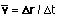The instantaneous velocity is given by a similar formula, with the condition that a very small time interval is used to measure the displacement.

A similar formula gives the average acceleration:Again, the instantaneous acceleration is found by measuring the change in velocity over a small time interval.

### The constant acceleration equations

When the acceleration is constant, we can write out four equations relating the displacement, initial velocity, velocity, acceleration, and time for each dimension. Like the 1D equations, these apply under the following conditions:

1. the acceleration is constant
2. the motion is measured from t = 0
3. the equations are vector equations, but the variables are not normally written in bold letters. The fact that they are vectors comes in, however, with positive and negative signs.

If we focus on two dimensions, we get four equations for the x direction and four more for the y direction. The four x equations involve only the x-components, while the four y equations involve only the y-components.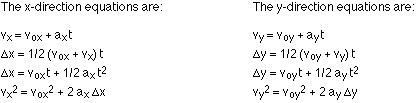One thing to notice is that the time, t, is the only thing that doesn't involve an x or a y. This is because everything else is a vector (or a component of a vector, if you'd rather look at it that way), but time is a scalar. Time is the one thing that can be used directly in both the x and y equations; everything else (displacement, velocity, and acceleration) has to be split into components.

### This is important!

Something that probably can't be emphasized enough is that even though an object may travel in a two-dimensional path (often following a parabola, in the standard case of an object moving under the influence of gravity alone), the motion can always be reduced to two independent one-dimensional motions. The x motion takes place as if the y motion isn't happening, and the y motion takes place independent of whatever is happening in the x direction.

One good example of this is the case of two objects (e.g., baseballs) which are released at the same time. One is dropped so it falls straight down; the other is thrown horizontally. As long as they start at the same height, both objects will hit the ground at the same time, no matter how fast the second one is thrown.

### What we're ignoring

We will generally neglect the effect of air resistance in most of the problems we do. In some cases that's just fine. In other cases it's not so fine. A feather and a brick dropped at the same time from the same height will not reach the ground at the same time, for example. This has nothing to do with the weight of the feather compared to the brick. It's simply air resistance; if we took away all the air and dropped the feather and brick, they would hit the ground at exactly the same time.

So, remember that we're often analyzing ideal cases, especially this early in the semester. In reality, things might be a little different because of factors we're neglecting at the moment.

### An example

Probably the simplest way to see how to apply these constant acceleration equations is to work through a sample problem. Let's say you're on top of a cliff, which drops vertically 150 m to a flat valley below. You throw a ball off the cliff, launching it at 8.40 m/s at an angle of 20° above the horizontal.
(a) How long does it take to reach the ground?
(b) How far is it from the base of the cliff to the point of impact?

It's a good idea to be as systematic as possible when it comes to analyzing the situation. Here's an example of how to organize the information you're given. First, draw a diagram.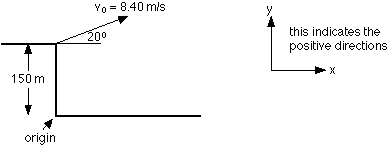Then set up a table to keep track of everything you know. It's important to pick an origin, and to choose a coordinate system showing positive directions. In this case, the origin was chosen to be the base of the cliff, with +x being right and +y being up. You don't have to choose the origin or the positive directions this way. Pick others if you'd like, and stick with them (an origin at the top of the cliff, and/or positive y-direction down would be two possible changes).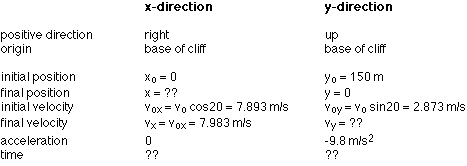Now that everything's neatly organized, think about what can be used to calculate what. You know plenty of y-information, so we can use that to find the time it takes to reach the ground. One way to do this (definitely not the only way) is to do it in two steps, first calculating the final velocity using the equation: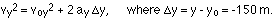This gives vy2 = 2.8732 + 2 (-9.8) (-150) = 2948.3 m2 / s2 . Taking the square root gives: vy = +/- 54.30 m/s.

Remember that the square root can be positive or negative. In this case it's negative, because the y-component of the velocity will be directed down when the ball hits the ground.

Now we can use another equation to solve for time: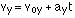So, -54.30 = 2.873 - 9.8 t, which gives t = 5.834 seconds. Rounding off, the ball was in the air for 5.83 s.

We can use this time for part (b), to get the distance traveled in the x-direction during the course of its flight. The best equation to use is: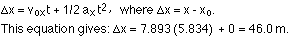So, from the base of the cliff to the point of impact is 46.0 m.

### A point about symmetry

At some point in its flight, the ball in the example above returned to the level of the top of the cliff (you threw it from the top of the cliff, it went up, and on its way down it passed through a point the same height off the ground as the top of the cliff). What was the ball's velocity when it passed this height? Its speed will be the same as the initial speed, 8.40 m/s, and its angle will be the same as the launch angle, only measured below the horizontal.

This is not just true of the initial height. At every height the ball passes through on the way up, there is a mirror-image point (at the same height, with the same speed, and the same angle, just down rather than up) on the downward part of the path.